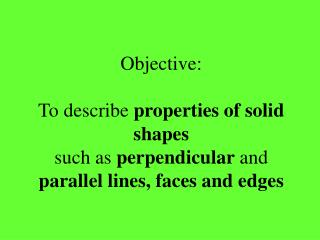DownloadDownload PresentationObjective: To describe properties of solid shapes such as perpendicular and parallel lines, faces and edges

# Objective: To describe properties of solid shapes such as perpendicular and parallel lines, faces and edges

Télécharger la présentation## Objective: To describe properties of solid shapes such as perpendicular and parallel lines, faces and edges

- - - - - - - - - - - - - - - - - - - - - - - - - - - E N D - - - - - - - - - - - - - - - - - - - - - - - - - - -
##### Presentation Transcript

1. Objective:To describe properties of solid shapessuch as perpendicular and parallel lines, faces and edges

2. PART 1SOLID SHAPES

3. Two-dimensional Shapes (2D) • These shapes are flat and can only be drawn on paper. • They have two dimensions – length and width. • They are sometimes called plane shapes.

4. Three-dimensional Shapes (3D) • These shapes are solid or hollow. • They have three dimensions – length, width and height.

5. Face • Part of a shape that is flat.(Or curved) • E.g. A cube has 6 of these.

6. Edge • The line where two faces meet. • E.g. A cube has 12 of these.

7. Vertex (Vertices) • The place where three or more edges meet. • This pyramid has 4 of these.

8. Parallel • These type of lines stay the same distance apart for their whole length. They do not need to be straight or the same length.

9. Perpendicular • A line that is drawn in a right angle to another line . • In solid shapes edges could be at a right angle to one another. • Faces could also be at right angles to one another.

10. Cube • A three-dimensional shape which has 6 square faces all the same size. Some faces parallel Some edges parallel Some faces perpendicular Some edges perpendicular

11. Cuboid • A three-dimensional shape which has 6 rectangular faces. Some faces parallel Some edges parallel Some faces perpendicular Some edges perpendicular

12. Sphere • A perfectly round three-dimensional shape, like a ball. It has only one curved face. No parallel faces or edges No perpendicular faces or edges

13. Hemisphere • A three-dimensional shape that is half a sphere. No parallel faces or edges No perpendicular faces or edges

14. Cone • A three dimensional shape with a circle at its base and a pointed vertex. No parallel faces or edges No perpendicular faces or edges

15. Cylinder • A three-dimensional shape with circular ends of equal size. Some faces parallel Some edges parallel Some faces perpendicular No edges perpendicular

16. Pyramid • A three-dimensional shape which has a polygon for its base and triangular faces which meet at one vertex. • E.g. Triangular pyramid • Square pyramid • Hexagonal pyramid • Pentagonal pyramid

17. Square base pyramid No faces parallel Some edges parallel No faces perpendicular Some edges perpendicular

18. Prism • A three dimensional shape that has the same cross-section all along its length.

19. Written task SOME PARALLEL FACES NO PARALLEL FACES Cube Cuboid SOME PERPENDICULAR FACES Cone Square based- Pyramid Sphere Hemispere NO PERPENDICULAR FACES

20. Written task SOME PARALLEL EDGES NO PARALLEL EDGES Cube Cuboid Square-based pyramid SOME PERPENDICULAR EDGES Sphere Hemisphere Cone NO PERPENDICULAR EDGES

21. PART 2PLANE SHAPES

22. Polygons Two-dimensional shapes that have sides made from straight lines. • E.g. triangles squares hexagons

23. Quadrilaterals • Any two-dimensional shapes with four straight sides. • E.g. square rhombus rectangle trapezium kite

24. Rectangle • A four sided two-dimensional shape with two pairs of parallel sides that meet at right angles.

25. Kite • A two-dimensional shape with two shorter sides of equal length and two longer sides of equal length.

26. Rhombus • A two-dimensional four sided shape with opposite sides that are parallel and all the sides are the same length.

27. Trapezium • A two-dimensional shape with four sides.One pair of sides is parallel with one side longer than the other.

28. Pentagon • A two-dimensional shape with five straight sides and five angles.

29. Hexagon • A two-dimensional shape with 6 straight sides and 6 angles.

30. Octagon • A two-dimensional shape with 8 straight sides and 8 angles.

31. What do we call a shape with:7 Sides?9 Sides?10 Sides?

32. Circle • A round flat two-dimensional shape.

33. Square • Two dimensional shape with 4 sides of the same length and 4 90° angles.

34. Triangle • Two-dimensional shape with three straight sides and three angles. • Can you name 4 different kinds?